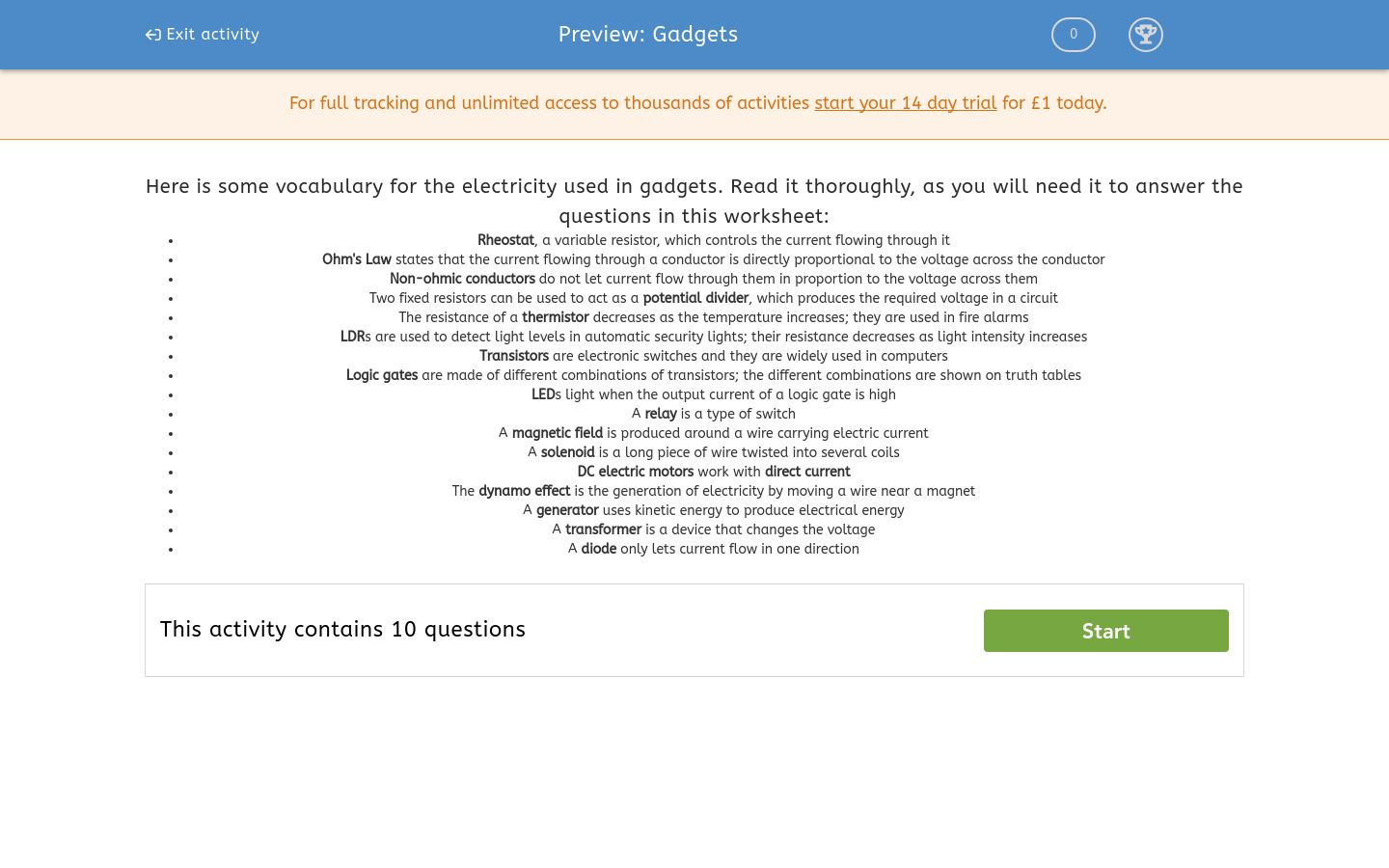### Affordable pricing from £10/month

In this worksheet, students explore the science used behind gadgets.Key stage:  KS 4

Curriculum topic:  Physics: Electricity

Curriculum subtopic:  Exploring Current, Resistance and Voltage Relationships

Difficulty level:### QUESTION 1 of 10

Here is some vocabulary for the electricity used in gadgets. Read it thoroughly, as you will need it to answer the questions in this worksheet:

• Rheostat, a variable resistor, which controls the current flowing through it
• Ohm's Law states that the current flowing through a conductor is directly proportional to the voltage across the conductor
• Non-ohmic conductors do not let current flow through them in proportion to the voltage across them
• Two fixed resistors can be used to act as a potential divider, which produces the required voltage in a circuit
• The resistance of a thermistor decreases as the temperature increases; they are used in fire alarms
• LDRs are used to detect light levels in automatic security lights; their resistance decreases as light intensity increases
• Transistors are electronic switches and they are widely used in computers
• Logic gates are made of different combinations of transistors; the different combinations are shown on truth tables
• LEDs light when the output current of a logic gate is high
• A relay is a type of switch
• A magnetic field is produced around a wire carrying electric current
• A solenoid is a long piece of wire twisted into several coils
• DC electric motors work with direct current
• The dynamo effect is the generation of electricity by moving a wire near a magnet
• A generator uses kinetic energy to produce electrical energy
• A transformer is a device that changes the voltage
• A diode only lets current flow in one direction

What does a rheostat do?

it splits up current

it controls current flowing through it

it works as a switch

What does Ohm's Law state?

the current flowing through a conductor is directly proportional to the voltage across the conductor squared

the current flowing through a conductor is inversely proportional to the voltage across the conductor

the current flowing through a conductor is directly proportional to the voltage across the conductor

How many fixed resistors can be used as a potential divider?

1

2

3

4

What happens to the resistance of a thermistor as the temperature increases?

increases

stays the same

decreases

What do LDRs detect?

light

sound

heat

What is a transistor?

electronic switch

light detector

controls current

Select the word from the options below which completes this sentence:

Logic gates are made of different combinations of _____________.

thermistors

rheostats

transistors

Where are the different combinations of transistors in logic gates shown?

in truth tables

in real tables

in fake tables

What type of energy does a generator use to produce electrical energy?

thermal (heat)

kinetic

sound

What does a transformer change?

current

voltage

charge

• Question 1

What does a rheostat do?

it controls current flowing through it
EDDIE SAYS
A rheostat controls current flowing through it.
• Question 2

What does Ohm's Law state?

the current flowing through a conductor is directly proportional to the voltage across the conductor
EDDIE SAYS
Ohm's Law states that the current flowing through a conductor is directly proportional to the voltage across the conductor.
• Question 3

How many fixed resistors can be used as a potential divider?

2
EDDIE SAYS
Two fixed resistors can be used as a potential divider.
• Question 4

What happens to the resistance of a thermistor as the temperature increases?

decreases
EDDIE SAYS
The resistance of a thermistor decreases as the temperature increases.
• Question 5

What do LDRs detect?

light
EDDIE SAYS
LDRs detect light.
• Question 6

What is a transistor?

electronic switch
EDDIE SAYS
A transistor is an electronic switch.
• Question 7

Select the word from the options below which completes this sentence:

Logic gates are made of different combinations of _____________.

transistors
EDDIE SAYS
Logic gates are made of different combinations of transistors.
• Question 8

Where are the different combinations of transistors in logic gates shown?

in truth tables
EDDIE SAYS
The different combinations of transistors in logic gates are shown in truth tables.
• Question 9

What type of energy does a generator use to produce electrical energy?

kinetic
EDDIE SAYS
A generator uses kinetic energy to produce electrical energy.
• Question 10

What does a transformer change?

voltage
EDDIE SAYS
A transformer changes voltage.
---- OR ----

Sign up for a £1 trial so you can track and measure your child's progress on this activity.

### What is EdPlace?

We're your National Curriculum aligned online education content provider helping each child succeed in English, maths and science from year 1 to GCSE. With an EdPlace account you’ll be able to track and measure progress, helping each child achieve their best. We build confidence and attainment by personalising each child’s learning at a level that suits them.

Start your £1 trial